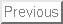Next: Introduction

Verification of Second-Order Sufficient
Optimality Conditions for Semilinear Elliptic
and Parabolic Control Problems

HANS D. MITTELMANN

Arizona State University, Department of Mathematics, Tempe, AZ 85287-1804, USA, mittelmann@asu.edu

Abstract: We study optimal control problems for semilinear parabolic equations subject to control constraints and for semilinear elliptic equations subject to control and state constraints. We quote known second-order sufficient optimality conditions (SSC) from the literature. Both problem classes, the parabolic one with boundary control and the elliptic one with boundary or distributed control, are discretized by a finite difference method. The discrete SSC are stated and numerically verified in all cases providing an indication of optimality where only necessary conditions had been studied before.

Key words: Elliptic and parabolic control problems, control and state constraints, second-order sufficient conditions, discretizations, optimization methods

Hans D. Mittelmann
2001-06-17